### Tests for Location

Subsections:

PROC UNIVARIATE provides three tests for location: Student’stest, the sign test, and the Wilcoxon signed rank test. All three tests produce a test statistic for the null hypothesis that the mean or median is equal to a given value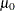against the two-sided alternative that the mean or median is not equal to. By default, PROC UNIVARIATE sets the value ofto zero. You can use the MU0= option in the PROC UNIVARIATE statement to specify the value of. Student’stest is appropriate when the data are from an approximately normal population; otherwise, use nonparametric tests such as the sign test or the signed rank test. For large sample situations, thetest is asymptotically equivalent to atest. If you use the WEIGHT statement, PROC UNIVARIATE computes only one weighted test for location, thetest. You must use the default value for the VARDEF= option in the PROC statement (VARDEF=DF). See Example 4.12.

You can also use these tests to compare means or medians of paired data. Data are said to be paired when subjects or units are matched in pairs according to one or more variables, such as pairs of subjects with the same age and gender. Paired data also occur when each subject or unit is measured at two times or under two conditions. To compare the means or medians of the two times, create an analysis variable that is the difference between the two measures. The test that the mean or the median difference of the variables equals zero is equivalent to the test that the means or medians of the two original variables are equal. Note that you can also carry out these tests by using the PAIRED statement in the TTEST procedure; see Chapter 103: The TTEST Procedure in SAS/STAT User's Guide. Also see Example 4.13.

#### Student’s t Test

PROC UNIVARIATE calculates thestatistic as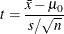where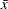is the sample mean,is the number of nonmissing values for a variable, andis the sample standard deviation. The null hypothesis is that the population mean equals. When the data values are approximately normally distributed, the probability under the null hypothesis of astatistic that is as extreme, or more extreme, than the observed value (the
-value) is obtained from thedistribution with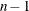degrees of freedom. For large, thestatistic is asymptotically equivalent to atest. When you use the WEIGHT statement and the default value of VARDEF=, which is DF, thestatistic is calculated as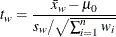where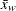is the weighted mean,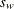is the weighted standard deviation, and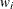is the weight forth observation. The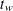statistic is treated as having a Student’sdistribution withdegrees of freedom. If you specify the EXCLNPWGT option in the PROC statement,is the number of nonmissing observations when the value of the WEIGHT variable is positive. By default,is the number of nonmissing observations for the WEIGHT variable.

#### Sign Test

PROC UNIVARIATE calculates the sign test statistic as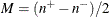where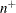is the number of values that are greater than, and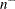is the number of values that are less than. Values equal toare discarded. Under the null hypothesis that the population median is equal to, the-value for the observed statistic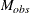is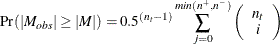where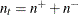is the number ofvalues not equal to.

Note: Ifandare equal, the-value is equal to one.

#### Wilcoxon Signed Rank Test

The signed rank statistic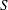is computed as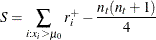where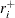is the rank of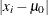after discarding values of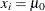, and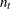is the number ofvalues not equal to. Average ranks are used for tied values.

If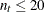, the significance ofis computed from the exact distribution of, where the distribution is a convolution of scaled binomial distributions. When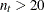, the significance ofis computed by treating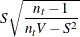as a Student’svariate with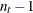degrees of freedom.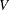is computed as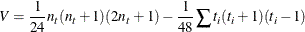where the sum is over groups tied in absolute value and where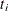is the number of values in theth group (Iman, 1974; Conover, 1980). The null hypothesis tested is that the mean (or median) is, assuming that the distribution is symmetric. Refer to Lehmann and D’Abrera (1975).